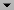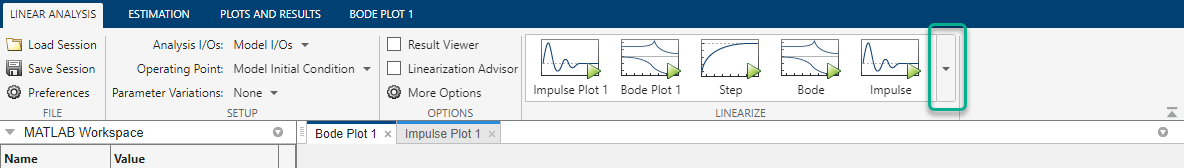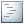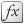Documentation

## Generate MATLAB Code for Linearization from Linear Analysis Tool

This topic shows how to generate MATLAB® code for linearization from the Linear Analysis Tool. You can generate either a MATLAB script or a MATLAB function. To programmatically reproduce a linearization result that you obtained interactively, you can use a generated MATLAB script. To perform multiple linearizations with systematic variations in your linearization configuration, you can use a generated MATLAB function.

To generate MATLAB code for linearization:

1. In the Linear Analysis Tool, on the Linear Analysis tab, interactively configure the analysis points, operating points, and parameter variations for linearization.

2. In the Linearize section, expand the gallery by clicking.3. In the gallery, depending on the type of code you want to create, click:

•`Script` — Generate a MATLAB script that uses your configured analysis points, operating points, and parameter variations. Select this option when you want to repeat the same linearization at the MATLAB command line.

•`Function` — Generate a MATLAB function that takes analysis points, operating points, and parameter variations as input arguments. Select this option when you want to perform multiple linearizations using different configurations (batch linearization). For more information on varying operating points and parameter when using the `linearize` function, see:

The software creates a MATLAB file that contains the generated code and opens the file in the MATLAB Editor.

4. In the MATLAB Editor, you can edit and save the file.

## Related Topics

##### SupportGet trial now